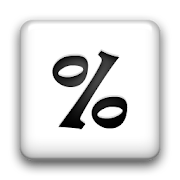# Percent Calculator + (AdFree) 1.4.4 APK    
4.8/5
6 Ratings
Developer
Current Version
1.4.4
Date Published
File Size
159k
Package ID
com.coinsoft.android.percentcalcpaid
Price
0.69
50+
Category
Android Apps
Genre
Tools

## APK Version History

Version
1.4.4 (Updated)
Architecture
All
Release Date
July 09, 2011
Requirement
Android 1.5 and up
Safe
••••This is the ad-free version of Percent Calculator +.

Percent Calculator + is a simple and easy to use percent calculator designed for anyone who wants to perform percentage calculations. Features include:

1. Percentage - Calculate percentage

2. Discount and Increment - Increase or decrease a value by a percentage amount.

3. Percent Of - Input two numbers to calculate the percentage of the first number based on the second number.

4. Before Discount / Mark Up - Calculate original value of a number before it has been reduced by a percentage.

5. Before Increment - Calculate original value of a number before it has been increased by a percentage.

6. Percent Change - Calculate the percentage change from one number to another number.

7. Tips Calculator - Calculate tips.